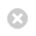Close

UCL Earth Sciences

Home# GEOL0046 Deep Earth & Planetary Modelling

The course aims to provide an understanding of key topics in modern techniques to simulate the deep Earth, with particular emphasis to atomistic computer simulations. Specific examples from current research will be presented.

Coordinator: Prof Dario Alfe

Module details
 Title Deep Earth and Planetary Modelling UG Code GEOL0046 Coordinator Prof Dario Alfe Other Contributors Dr Monica Pozzo Term 2 Credit 15 credits Written Exam Coursework 100% - 1,2,3,4 < 1000 words (25% each) Pre-Requisites None Maths & Stats Content and Requirement simple trigonometry, differentiation and integration of simple functions, logarithms Total Number of Hours of Student Work 188 hours Hours of Lectures/Seminars 20 hours Hours of Practicals/Problem Classes 20 hours Hours of Tutorials 0 Days of Fieldwork 0 Other None
Content
• Basic thermodynamic concepts and their relation to microscopic physics. 1st and 2nd law of thermodynamics. The direction of natural processes.
• Probability and its relation to Entropy. The partition function.
• Thermodynamic potentials: Helmholtz free energy, Gibbs free energy, Enthalpy. Equivalent formulations of the 2nd law.
• The Boltzmann and Gibbs distributions, canonical and gran-canonical averages, sampling methods.
• Calculating free energies with modern computational methods to simulate materials at high pressure and temperature.
• the Earth internal structure.
• earth's core composition, temperature and transport properties.
• phase diagrams and phase boundaries within the Earth.
• Practical examples, e.g. thermal expansion of materials. Hands on computer experiments.

#### AIMS

The course aims to provide an understanding of key topics in modern techniques to simulate the deep Earth, with particular emphasis to atomistic computer simulations. Lectures will be based on white board development of the underlying theory and computational methods. Practicals will be based on hands on computer experiments. Specific examples from current research will be presented.

#### Outcomes

Students will become familiar with the following concepts:

• Elements of thermodynamics and statistical physics
• Modern computational methods to simulate materials at high pressure and temperature.
• Phase diagrams.
• The Earth internal structure.
• Earth's core composition, temperature and transport properties.

#### Practical and transferable outcomes

• Use of computer simulations to calculate phase diagrams, both at zero and at finite temperature.
• Equation of states and how to use them to find thermodynamic stability of materials at high pressure and temperature.
• Comparison theory-experiment and critical evaluation of predictive power of theoretical simulation methods.
• Use of phase diagrams to interpret planetary bodies interiors.
• IT skills.